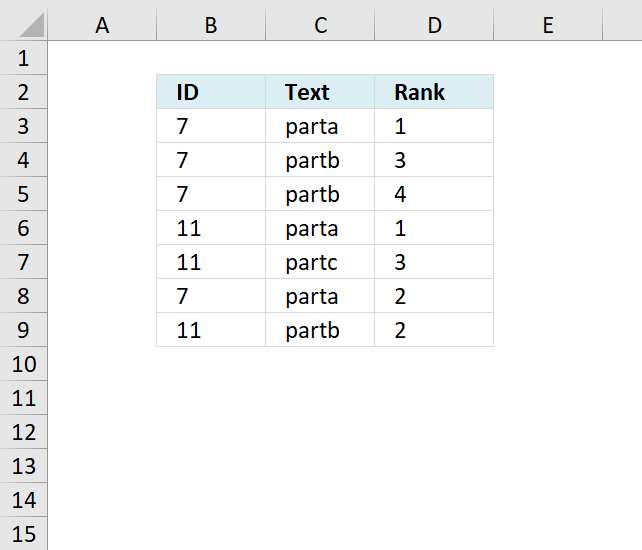Author: Oscar Cronquist Article last updated on November 18, 2018The following formula ranks text values in column C uniquely based on the category in column B.

Formula in D3:

=SUMPRODUCT((C3&ROW()>\$C\$3:\$C\$9&ROW(\$C\$3:\$C\$9))*(B3=\$B\$3:\$B\$9))+1

### Explaining formula in cell D3

#### Step 1 - Concatenate cell C3 and current row number

The ROW function returns the row number from a cell reference, if a cell ref is omitted then the row number of the current cell is returned.

The ampersand character & concatenates two values.

C3&ROW()

becomes

"parta"&3

and returns parta3.

#### Step - 2 Concatenate each cell in column C and corresponding row numbers row-wise

\$C\$3:\$C\$9&ROW(\$C\$3:\$C\$9)

becomes

{"parta";"partb";"partb";"parta";"partc";"parta";"partb"}&{3;4;5;6;7;8;9}

and returns

{"parta3";"partb4";"partb5";"parta6";"partc7";"parta8";"partb9"}

#### Step 3 - Compare value with array using the larger than sign

(C3&ROW()>\$C\$3:\$C\$9&ROW(\$C\$3:\$C\$9))

becomes

parta3>{"parta3";"partb4";"partb5";"parta6";"partc7";"parta8";"partb9"}

and returns

{FALSE;FALSE;FALSE;FALSE;FALSE;FALSE;FALSE}.

The number concatenated to each value makes it unique.

#### Step 4 - Compare categories with current cell

(B3=\$B\$3:\$B\$9)

becomes

7={7;7;7;11;11;7;11}

and returns

{TRUE;TRUE;TRUE;FALSE;FALSE;TRUE;FALSE}

#### Step - 5 Multiply arrays

(C3&ROW()>\$C\$3:\$C\$9&ROW(\$C\$3:\$C\$9))*(B3=\$B\$3:\$B\$9)

becomes

{FALSE;FALSE;FALSE;FALSE;FALSE;FALSE;FALSE}*{TRUE;TRUE;TRUE;FALSE;FALSE;TRUE;FALSE}

and returns

{0;0;0;0;0;0;0}

#### Step 6 - Sum values in array

SUMPRODUCT((C3&ROW()>\$C\$3:\$C\$9&ROW(\$C\$3:\$C\$9))*(B3=\$B\$3:\$B\$9))

becomes

SUMPRODUCT({0;0;0;0;0;0;0})

and returns 0.

#### Step 7 - Add 1

SUMPRODUCT((C3&ROW()>\$C\$3:\$C\$9&ROW(\$C\$3:\$C\$9))*(B3=\$B\$3:\$B\$9)) + 1

becomes

0 + 1 equals 1 in cell D3.